# Rhombus

A rhombus is a parallelogram with four congruent sides. The plural of rhombus is rhombi . (I love that word.)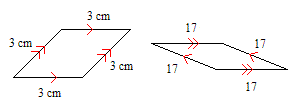The formula for the area of a rhombus is the same as the formula for a parallelogram:

$A=bh$ ,

where $b$ is the length of a base and $h$ is the height.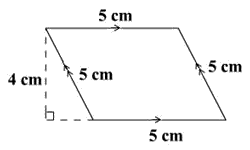$\text{Area}=5\cdot 4=20\text{\hspace{0.17em}}\text{\hspace{0.17em}}{\text{cm}}^{2}$ .

### Diagonals of a Rhombus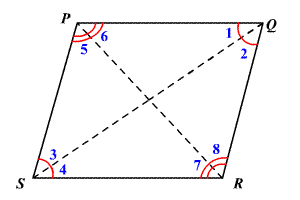If a parallelogram is a rhombus, then its diagonals are perpendicular.

For Example: If $PQRS$ is a rhombus, then $\stackrel{¯}{PR}\perp \stackrel{¯}{QS}$ .

If a parallelogram is a rhombus, then each diagonal bisects a pair of opposite angles.

For Example: If $PQRS$ is a rhombus, then

$\begin{array}{l}\angle 1\cong \angle 2,\text{\hspace{0.17em}}\text{\hspace{0.17em}}\angle 3\cong \angle 4,\text{\hspace{0.17em}}\text{\hspace{0.17em}}\angle 5\cong \angle 6,\text{\hspace{0.17em}}\text{and}\text{\hspace{0.17em}}\text{\hspace{0.17em}}\angle 7\cong \angle 8\end{array}$ .

Example 1:

In rhombus $ABCD$ , $m\angle ABC=2x-7\text{\hspace{0.17em}}\text{\hspace{0.17em}}\text{and}\text{\hspace{0.17em}}\text{\hspace{0.17em}}m\angle BCD=2x+3$ . Find $m\angle DAB.$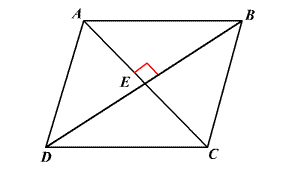In a rhombus, consecutive interior angles are supplementary. So,

$m\angle ABC+\text{\hspace{0.17em}}\text{\hspace{0.17em}}m\angle BCD=180°$ .

Substitute the measures and solve for $x$ .

$\begin{array}{l}2x-7+2x+3=180°\\ 4x=184\\ x=46\end{array}$

Use the value of $x$ to find $m\angle DAB.$

Each pair of opposite angles of a rhombus is congruent. So,

$m\angle DAB=m\angle BCD=2\left(46\right)+3=95°$ .

Example 2:

In rhombus $XYZW$ , $m\angle WYZ=63°$ . Find $m\angle YZV.$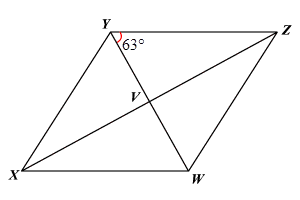The diagonals of a rhombus are perpendicular.

Use the Angle Sum Theorem.

$m\angle YZV+m\angle ZVY+m\angle VYZ=180°$

Substitute the measures and solve.

$m\angle YZV+90°+63°=180°$

$\begin{array}{c}m\angle YZV+153°=180°\\ m\angle YZV=27°\end{array}$